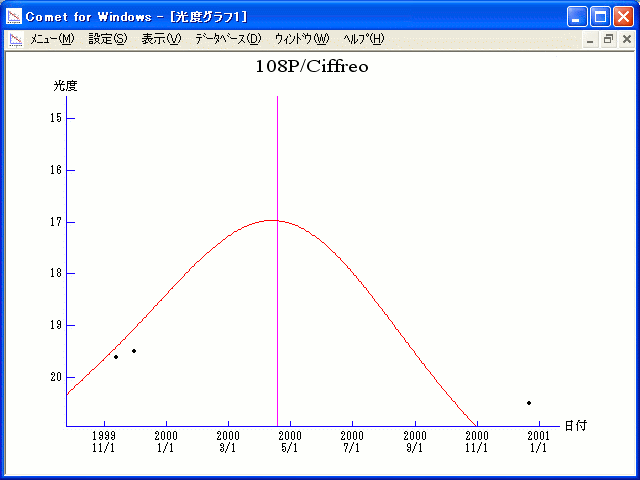# 108P/Ciffreo (2000)###Orbital Elements

```   The following improved orbital elements by Kenji Muraoka, are
from 102 observations  1985 to 1999, including 9 Planets, Moon
and 5 minor planets perturbations and non-gravitational effect
of style II.    The mean residual is +/- 0.93 arc seconds.

Epoch  =  2000 Apr.  6.0  TT       JDT = 2451640.5
T  =  2000 Apr. 18.39097       +/- 0.00230 (m.e.) TT
Peri. =  358.04249                +/- 0.00128
Node  =   53.72152                +/- 0.00007   (2000.0)
Incl. =   13.09288                +/- 0.00004
q  =    1.7133555              +/- 0.0000025 AU
e  =    0.5426166              +/- 0.0000029
a  =    3.7459937              +/- 0.0000066 AU
n  =    0.13594195             +/- 0.00000036
P  =    7.250                  +/- 0.0000192  years
A1  =   +0.243                  +/- 0.367
A2  =   -0.02479                +/- 0.00364
```

###Finding Charts###Magnitudes Graph

```        m1 = 9.0 + 5 log d + 25.0 log r
```##### The orbital elements are calculated by Kenji Muraoka. The charts are made with StellaNavigator Ver.2.0 for Windows (AstroArts / ASCII). The magnitudes graphs are made with Comet for Windows.